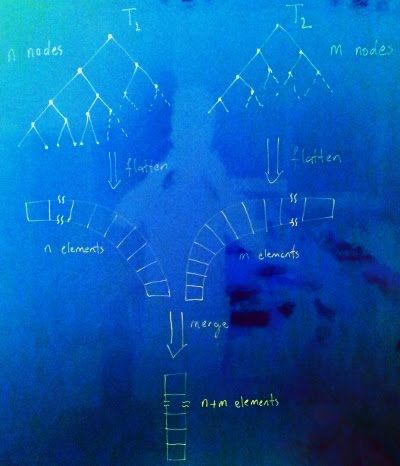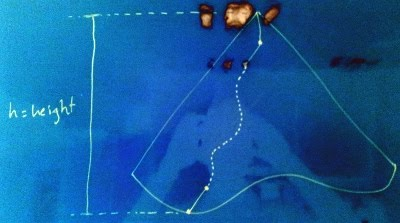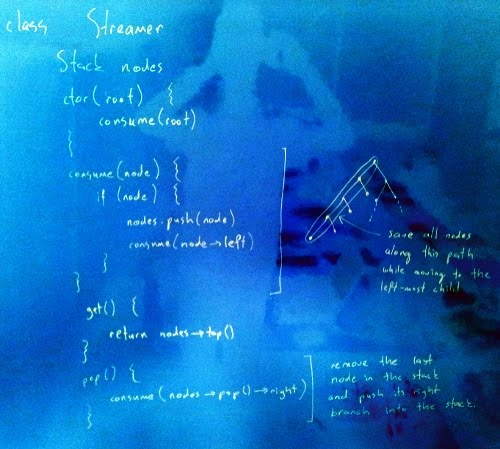Merging Two Binary Search Trees in O(logn) Space
posted at November 30, 2012 with tags algorithm, cpp

In a recent coding interview, I was asked to merge two Binary Search Trees (BSTs) without modifying them. This was the first time I was exposed to this question and for an hour or so I tried to come up with a linear time algorithm of O(1) space complexity. The defeat was inevitable. (To tell the truth, if a hint would be given like O(m+n) storage is allowed, I could easily figure out the solution without breaking a sweat.) As usual, I could not sleep that night and scratched dozens of papers to come up with a better solution. But as night passed, I started to realize the fact that the space complexity is lower bounded by the tree height. This blog post is set to keep a cyber-record of this pursuit.

# Related Work

You can first start by checking the Google results. But, as usual, I am kind enough to provide you a tl;dr version: In order to merge two BSTs of size m and n nodes, there are a couple of common approaches of fixed O(m+n) processing time and space complexity, some of which are listed as follows.

• Insert given BSTs into a newly created BST.
• Create an array of elements of the first BST in sorted order, and use this array to merge the results to a new BST while traversing the second BST.

Further exposure to the higher doses of the problem is available through this and this StackOverflow threads.

# On Complexity

You will definitely need an O(m+n) processing complexity to visit each node, that’s for sure. But what about O(m+n) space complexity? It means that you need to store one (or both) of the given trees in a vector in order to proceed with the merge. As it will turn out in the following paragraphs, actually space complexity is lower-bounded by the height of the tree, that is, O(h), where h=logn for a balanced tree.

# The Trick

In its most basic form, we flatten both trees into two separate vectors. Next, we consume one element at a time from either of the trees with the smallest element. This scheme deserves a figure of its own.It is certain that we effectively don’t need the whole elements of a tree packed into a single vector at once. At each step, what we ask for is the next smallest element. That is, we just need a stream of nodes traversed in-order.

Let’s further investigate the possibility of implementing a stream of nodes. In order to consume the elements of a binary search tree in sorted order, we need to traverse the tree in left-center-right node order. Assume that we have below traversal function. (Yes, it is in C++ and I shamelessly used templates.)

``````template <class T>
void traverse(Node<T>* root, queue<T>& items) {
if (root) {
traverse(root->left());
items.push(root->data());
traverse(root->right());
}
}``````

What if I can suspend the execution at any point in time while pushing the data to a queue? In that case, what would be the maximum possible height of a recursive `traverse()` call stack? I know you like figures, so I took another photo of the board.That is, the maximum call stack depth of a `traverse()` recursion is upper-bounded by the tree height. Coupled with the fact that successive `traverse()` calls are sufficient to consume the nodes of a tree in sorted order, we should be able to stream the nodes of tree with at most O(logn) node pointers.

# Streaming Nodes

Since actual traverse call stack is bounded, we can emulate the recursive traverse using a stack of the nodes traversed so far from the root. The outline of the streaming algorithm is as follows.# The Prestige

Now we can stream a tree in sorted order using at most O(logn) storage. The rest is easy: Stream both trees and merge them while streaming.

# The Code

I implemented a streamer (`NodeStream`), stream merger (`MergeNodeStream`), and a vector merger (`MergeNodeVector`) in C++ and Scala. Code is accessible through this Gist. You can also find implementations of the algorithm in C++ using Boost Coroutines and Haskell written by Remko.